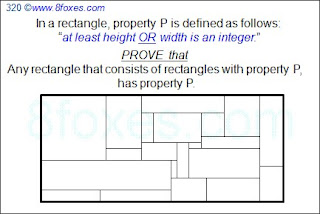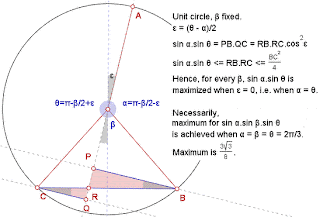Sunday, January 23, 2011

Fox 325

We've been carried away with very hard problems recently. Let's have a simple reality check with this classic. But, please try solving this without any words :)

Sunday, January 16, 2011

Fox 320 - SolutionsThis fox has been discussed extensively. See here...

1. Calculus by Bleaug

He says: "I spotted this problem in a Paul Halmos book "Problems for mathematicians, young and old, 1991", actually in a French translation. He provided the solution below (reformulated by myself) as being proposed by Hugh Montgomery in 1985 in some Math Conference."2. Checkerboard Solution by Rochberg and Stein:

1. Call sub rectangles as TILEs.

2. Start from the lower left corner of the overall rectangle (let's call this point as the origin)Draw horizontal and vertical lines separated by 1/2=0.5 units starting from the origin.

3. This will create 0.5 x 0.5 squares (and possibly rectangles around 2 edges -top and/or RHS)

4. Color the first square (that has the origin as one of its corners) as black the next one as white, and so on to generate a checkerboard-look.

5. Each TILE will have equal areas of black and white (Why?)

6. Therefore the overall rectangle will have equal areas of black and white.

7. So overall rectangle has at least one integer side.

H means the horizontal side is integer, and V means that the vertical side is integer. a1 is the square at the origin. There could have been a smarter combination, but this simple one illustrates the process clearly. This looks like the closest geometric solution we can get -at this time.When there's hardly no day nor hardly no night
There's things half in shadow and halfway in light

3. Induction by Robinson
1. Assume that each H-tile has a width of 1, and each V-tile has a height of 1. Note that any rectangle can be converted this way without distorting the original problem. (This may increase the number of tiles significantly though)

2. Chose any H-tile, say T(0). (If there is no H-tile, then the result is immediate)

3. If there are H-tiles whose lower border shares a segment with T(0)'s upper border, choose one and call it T(1).

4. Otherwise only V-tiles share this border. In this case, we can expand T(0) upward 1 unit. This does not increase the number of H-tiles. Also, the cut V-tiles still have height 1. (They are still V-tiles)

5. Continue expanding T(0) until either the top of the rectangle is reached or a choice of an adjacent H-tile T(1) is possible.

6. Then repeat the same process from T(1). (Continue upward similarly from T(1) to get T(2), and so on...)

7. This will result in a chain of T(0), T(1), T(2), ... , T(m).

8. Starting from T(0) again, work downward similarly to obtain a bigger chain:
T(-n), T(1-n), ... , T(0), T(1), ... , T(m-1), T(m) of H-tiles stretching from bottom to top.

9. Remove these tiles and slide the rest together to get a rectangle with fewer H-tiles.

10. Induction applied to this smaller rectangle yields the result for the original rectangle.

Sunday, January 9, 2011

Fox 15

This ancient fox was the general case of Fox 187, which was successfully-solved. And now, Bleaug claims that this has been solved, too. Let's see if we'll be able to confirm his claim.Friday, January 7, 2011

Fox 319 - Solutions

Below are 2 distinct solutions for Fox 319...
A solution based on symmetry by Bleaug:Trigonometry and calculus by Six:

x + y + z = 360 and sinx*siny*sinz

-> f(x,y) = sin(x)*sin(y)*sin(360-x-y)
-> f(x,y) = sin(x)*sin(y)*-sin(x+y)

Now to find the critical points of f(x,y), we just need to find the partial derivative with respect to x and y and solve for 0.

f_x(x,y) =

sin(x)sin(y)(-cos(x+y)-cos(x)sin(y)sin(x+y)

solve for 0.

sin(x)sin(y)(-cos(x+y)-cos(x)sin(y)sin(x+y)=0

-> tan(x) = -tan(x+y)

Since the function is symmetric, we should get the same partial derivative for y.

-> tan(y) = -tan(y+x)

-> tan(x)=tan(y)

-> x = y or they are opposites. However, if they are opposites, the original function just becomes 0. Thus, x = y.

Now substitute in x for y in the original equation and find its critical points.

Eventually, you will get sin(3x)=0

x = 120 degrees
y = 120 degrees
z = 120 degrees

http://www.8foxes.com/

Monday, January 3, 2011

Fox 324

Before starting to publish received solutions for earlier foxes, let's start with a new one. Here, "random selection" means that points P and Q are uniformly distributed along 2 parallel sides.
Ahh, yes, expected value of the "ratio of" the bigger area to the smaller one is asked - obviously. Let's give some leeway to minor mistakes in our life. "Perfect" may sometimes be boring!

Saturday, January 1, 2011

Happy new year everybody!A new year,
a new beginning,
An unheard adventure - even it makes you late for dinner,
a new beginning.

Cold air that you breath in,
A warm shoulder that you can lean
A promise you've given to yourself - one more time,
a new beginning.

A morning sky painted in the East,
A colorful sunset as it fills the West,
Bread, cheese, water - and the rest,
a new beginning.